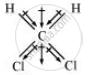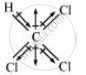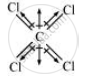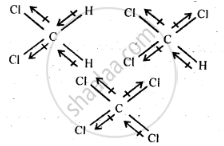Share

# Which One of the Following Has the Highest Dipole Moment? - Chemistry

#### Question

Which one of the following has the highest dipole moment?

(i) CH2Cl2

(ii) CHCl3

(iii) CCl4

#### Solution 1

1)Dichlormethane (CH2Cl2)

μ = 1.60D

2)Chloroform (CHCl3)

μ = 1.08D

3)Carbon tetrachloride (CCl4)

μ = 0D

CCl4 is a symmetrical molecule. Therefore, the dipole moments of all four C−Cl bonds cancel each other. Hence, its resultant dipole moment is zero.

As shown in the above figure, in CHCl3, the resultant of dipole moments of two C−Cl bonds is opposed by the resultant of dipole moments of one C−H bond and one C−Cl bond. Since the resultant of one C−H bond and one C−Cl bond dipole moments is smaller than two C−Cl bonds, the opposition is to a small extent. As a result, CHCl3 has a small dipole moment of 1.08 D.

On the other hand, in case of CH2Cl2, the resultant of the dipole moments of two C−Cl bonds is strengthened by the resultant of the dipole moments of two C−H bonds. As a result, CH2Cl2 has a higher dipole moment of 1.60 D than CHCl3 i.e., CH2Cl2 has the highest dipole moment.

Hence, the given compounds can be arranged in the increasing order of their dipole moments as:

CCl4 < CHCl3 < CH2Cl2

#### Solution 2

The three dimensional structures of the three compounds along with the direction of dipole moment in each of their bonds are given below:CCl4 being symmetrical has zero dipole moment. In CHCl3, the resultant of two C – Cl dipole moments is opposed by the resultant of C – H and C – Cl bonds. Since the dipole moment of latter resultant is expected to be smaller than the former, CHCl3 has a finite dipole (1.03 D) moment.
In CH2CI2, the resultant of two C – Cl dipole moments is reinforced by resultant of two C – H dipoles, therefore, CH2CI2 (1 .62 D) has a dipole moment higher than that of CHCl3. Thus, CH2CI2 has highest dipole moment.

Is there an error in this question or solution?